### IMO Shortlist 2008 problem G5

Kvaliteta:
Avg: 0,0
Težina:
Avg: 8,0
Let$k$ and$n$ be integers with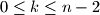$0\le k\le n - 2$. Consider a set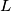$L$ of$n$ lines in the plane such that no two of them are parallel and no three have a common point. Denote by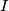$I$ the set of intersections of lines in$L$. Let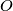$O$ be a point in the plane not lying on any line of$L$. A point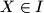$X\in I$ is colored red if the open line segment$OX$ intersects at most$k$ lines in$L$. Prove that$I$ contains at least$\dfrac{1}{2}(k + 1)(k + 2)$ red points.

Proposed by Gerhard Woeginger, Netherlands
Izvor: Međunarodna matematička olimpijada, shortlist 2008